## Usage

# S4 method for CalibratedAges,missing
plot(
x,
calendar = getOption("ananke.calendar"),
density = TRUE,
interval = TRUE,
level = 0.954,
sort = TRUE,
decreasing = TRUE,
main = NULL,
sub = NULL,
axes = TRUE,
frame.plot = FALSE,
ann = graphics::par("ann"),
panel.first = NULL,
panel.last = NULL,
col.density = "grey",
...
)

# S4 method for CalibratedSPD,missing
plot(
x,
calendar = getOption("ananke.calendar"),
main = NULL,
sub = NULL,
ann = graphics::par("ann"),
axes = TRUE,
frame.plot = FALSE,
panel.first = NULL,
panel.last = NULL,
...
)

## Arguments

x

A CalibratedAges or CalibratedSPD object.

calendar

A TimeScale object specifying the target calendar (see calendar()).

density

A logical scalar: should density be drawn?

interval

A logical scalar: should highest density region be drawn?

level

A length-one numeric vector giving the confidence level. Only used if interval is TRUE.

sort

A logical scalar: should the data be sorted?

decreasing

A logical scalar: should the sort order be decreasing? Only used if sort is TRUE.

main

A character string giving a main title for the plot.

sub

A character string giving a subtitle for the plot.

axes

A logical scalar: should axes be drawn on the plot?

frame.plot

A logical scalar: should a box be drawn around the plot?

ann

A logical scalar: should the default annotation (title and x and y labels) appear on the plot?

panel.first

An an expression to be evaluated after the plot axes are set up but before any plotting takes place. This can be useful for drawing background grids.

panel.last

An expression to be evaluated after plotting has taken place but before the axes, title and box are added.

col.density, col.interval

A specification for the plotting colors.

...

Other graphical parameters may also be passed as arguments to this function.

## Value

plot() is called it for its side-effects: it results in a graphic being displayed. Invisibly returns x.

Other radiocarbon tools: F14C, c14_calibrate(), c14_combine(), c14_curve(), c14_ensemble(), c14_spd(), c14_uncalibrate(), rec_plot

N. Frerebeau

## Examples

## Calibrate a single date
cal <- c14_calibrate(300, 20)
plot(cal, panel.first = graphics::grid())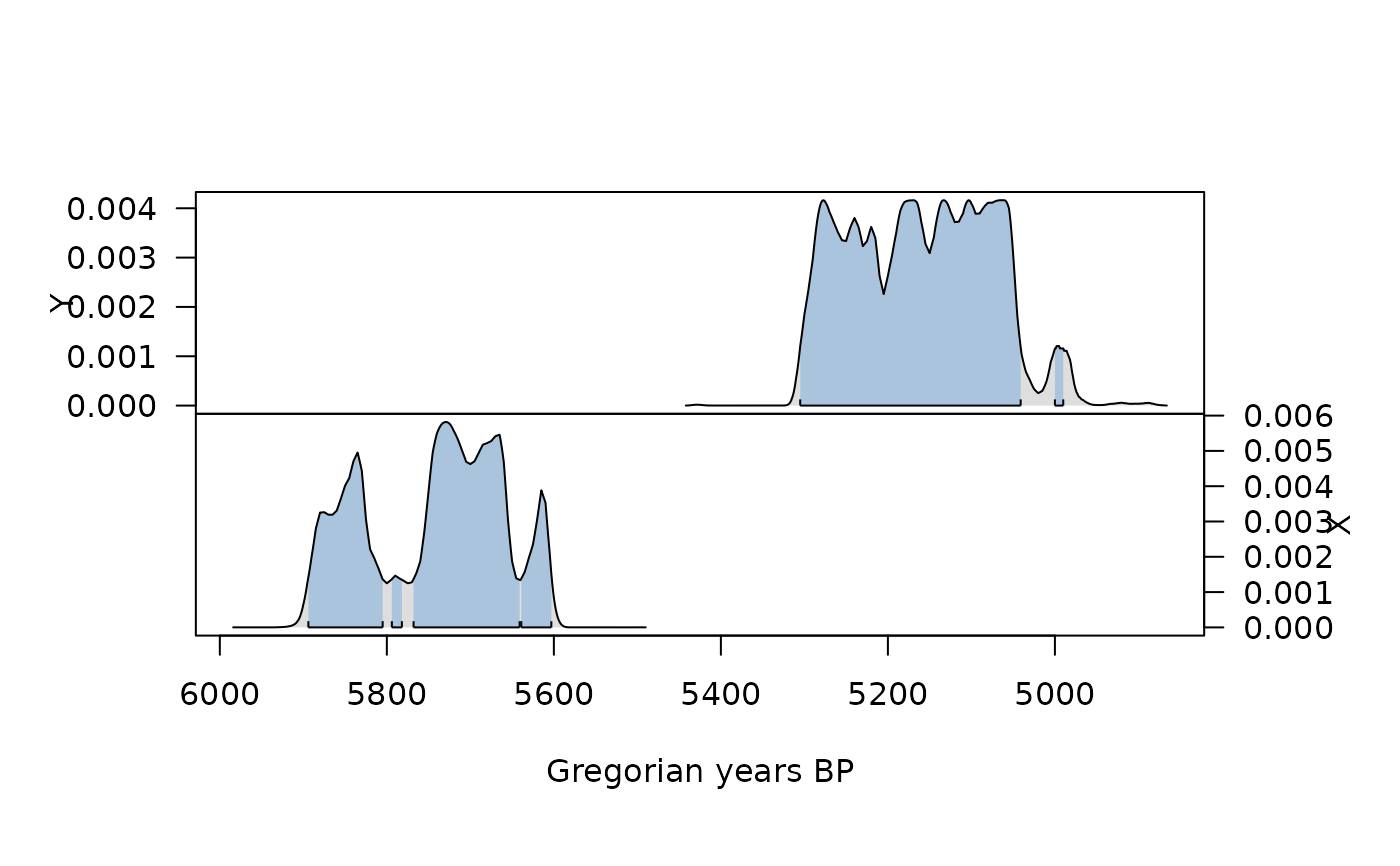## Calibrate multiple dates
cal <- c14_calibrate(
ages = c(5000, 4500),
errors = c(45, 35),
names = c("X", "Y")
)
plot(cal, calendar = BP(), panel.first = graphics::grid())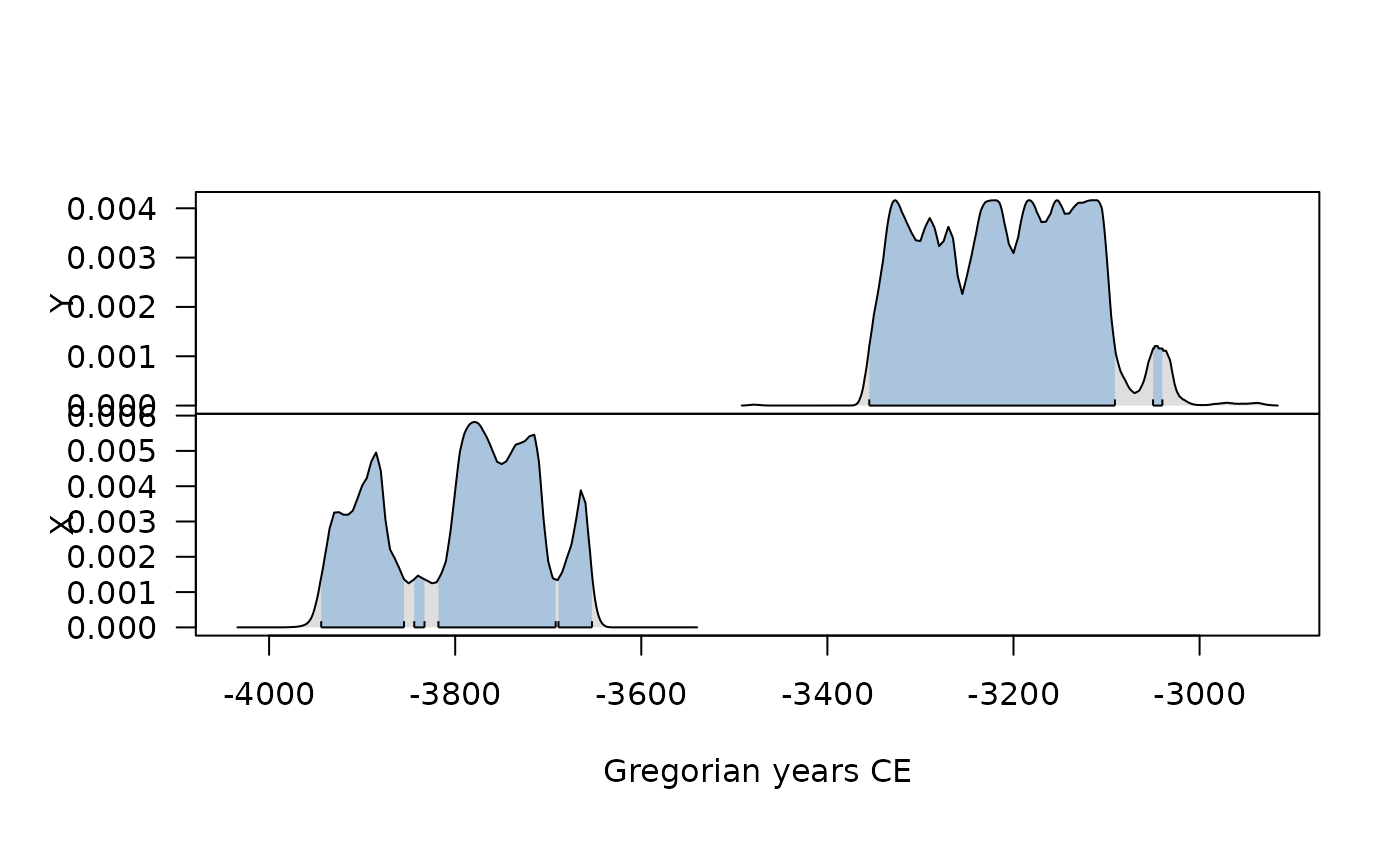plot(cal, interval = FALSE)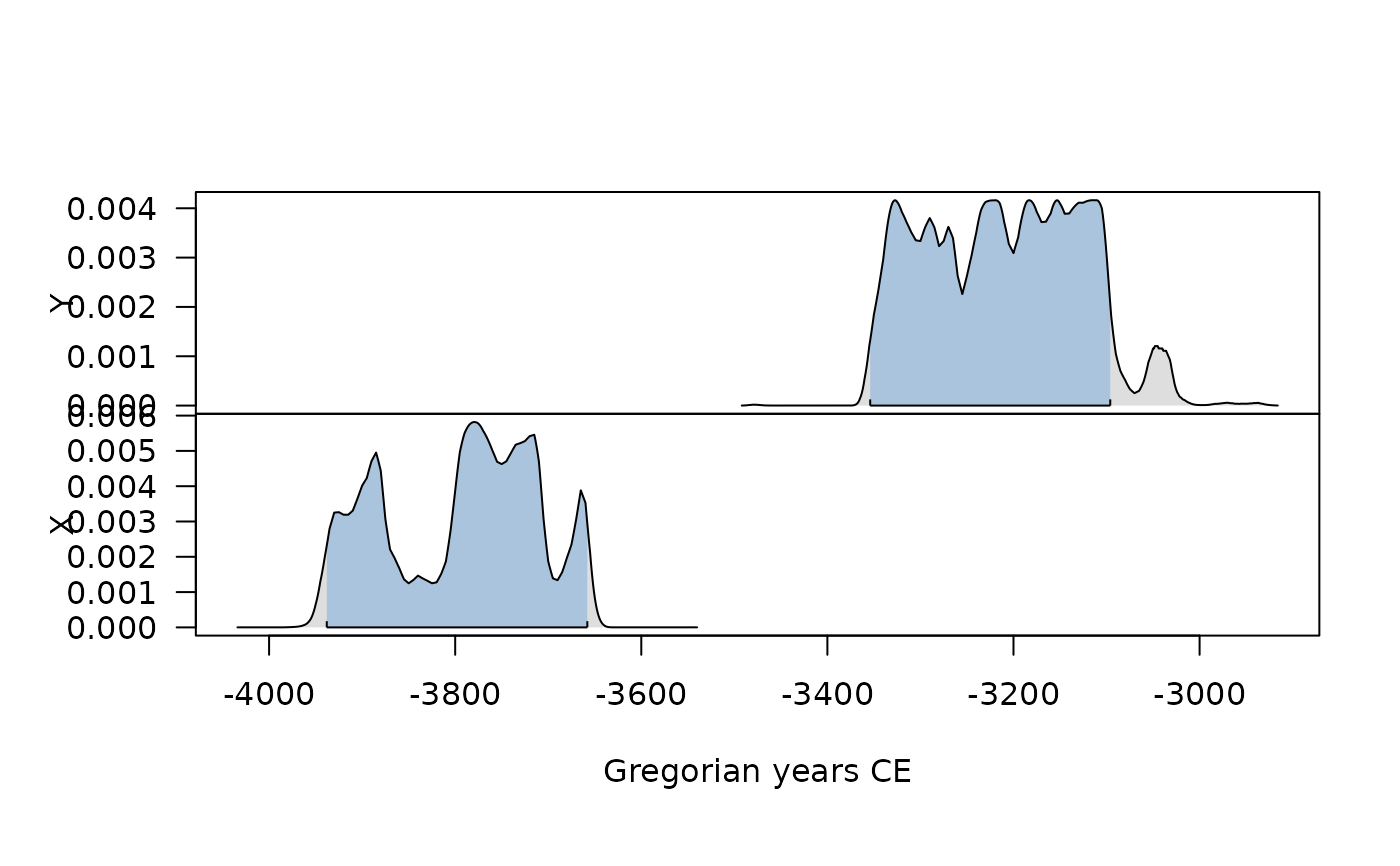plot(cal[, 1, ], col.interval = "red")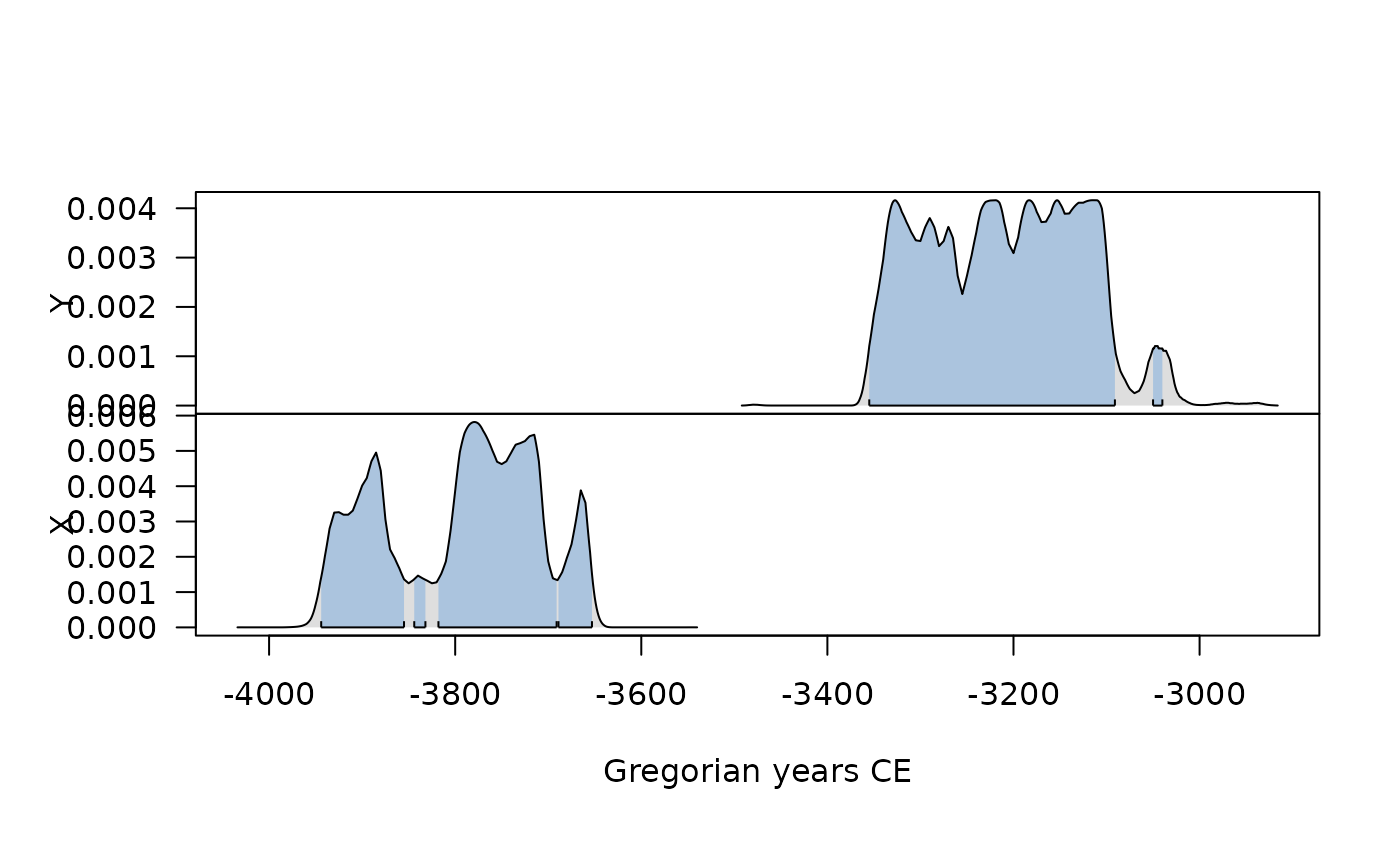plot(cal, density = FALSE, level = 0.68, lwd = 5)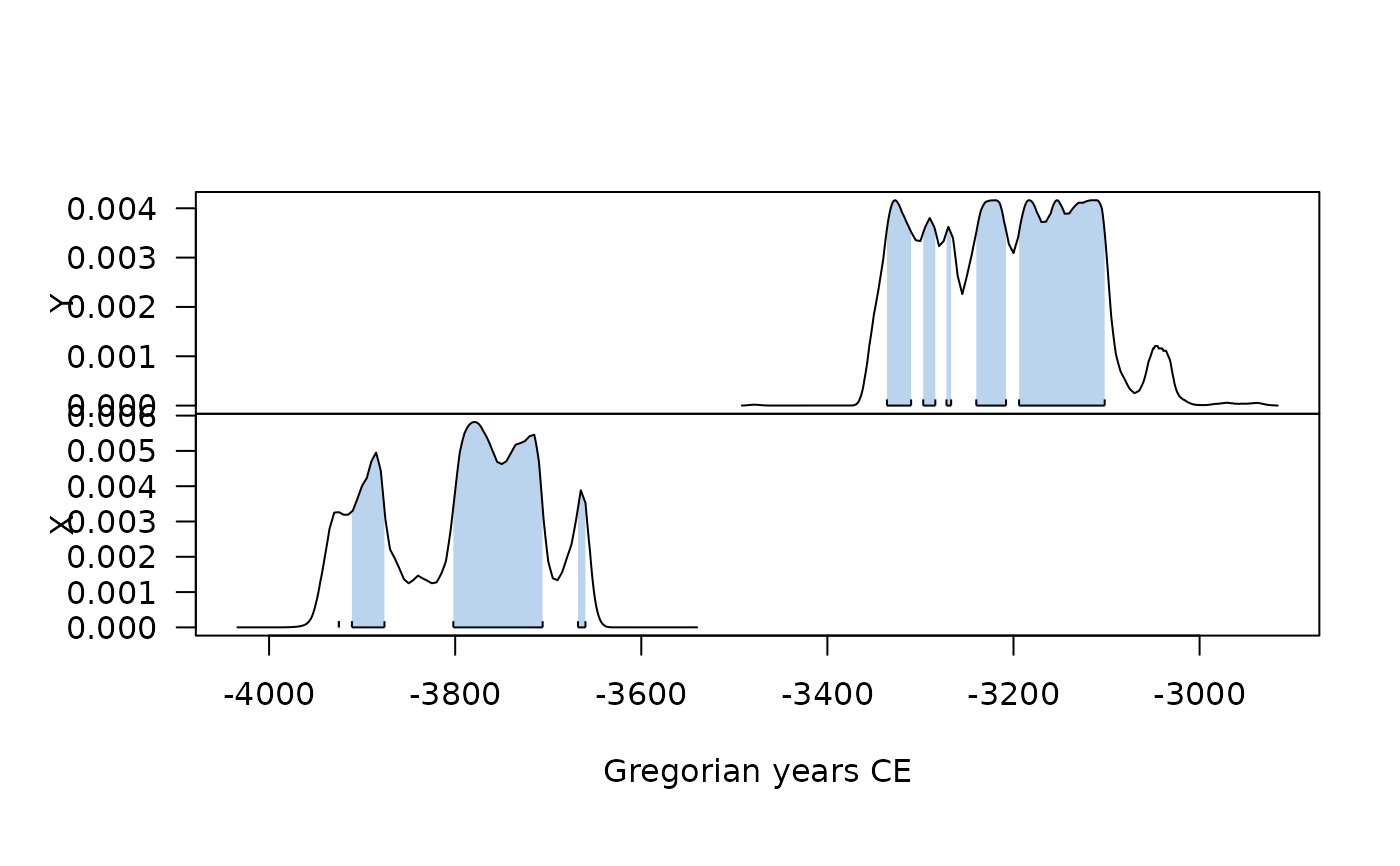plot(cal, density = FALSE, level = 0.95, lwd = 5)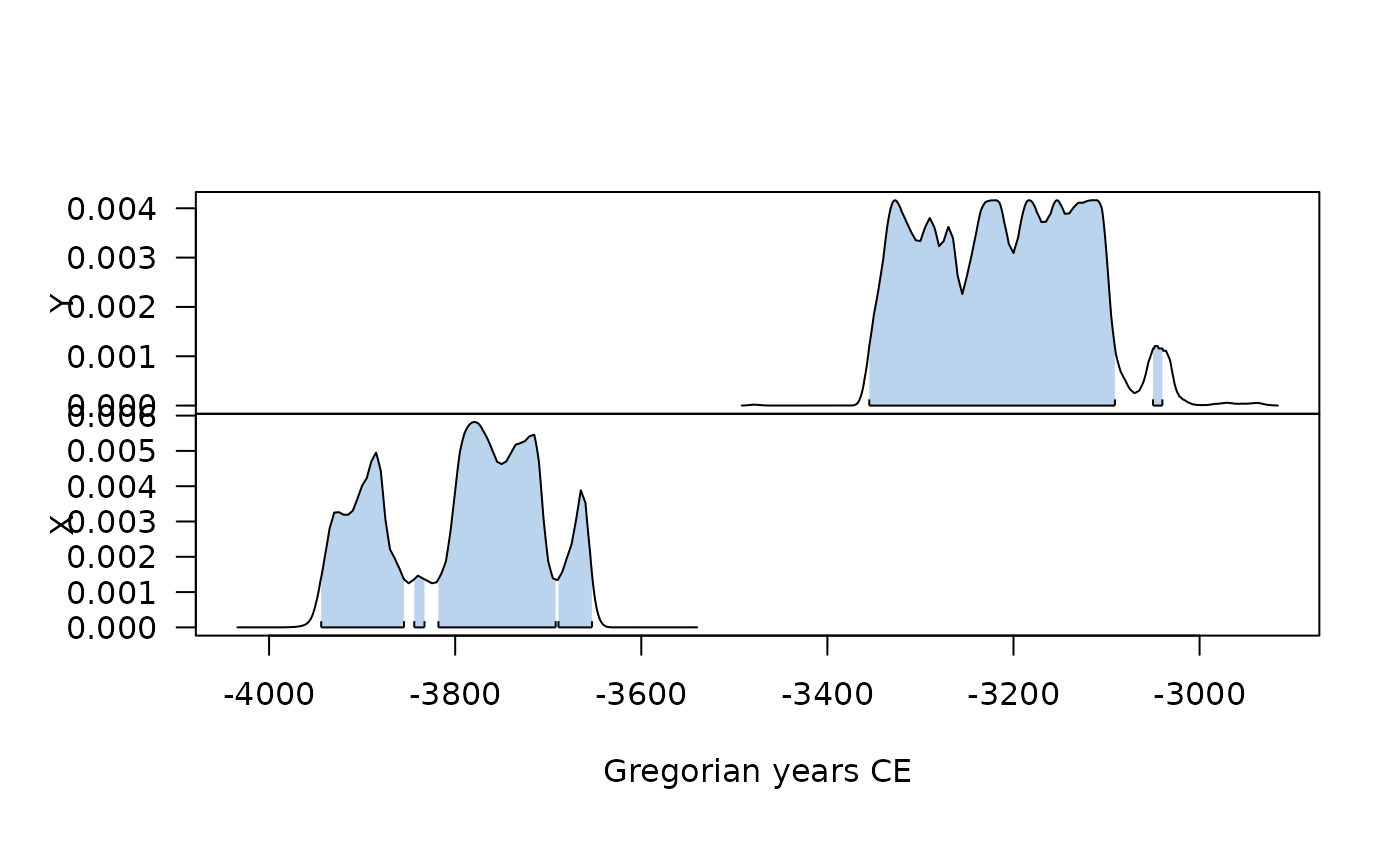# \donttest{
## Out of 14C range?
out <- c14_calibrate(130, 20)
#> Warning: Date “X1” may extent out of range.
plot(out)
#> Warning: Date “X1” may extent out of range.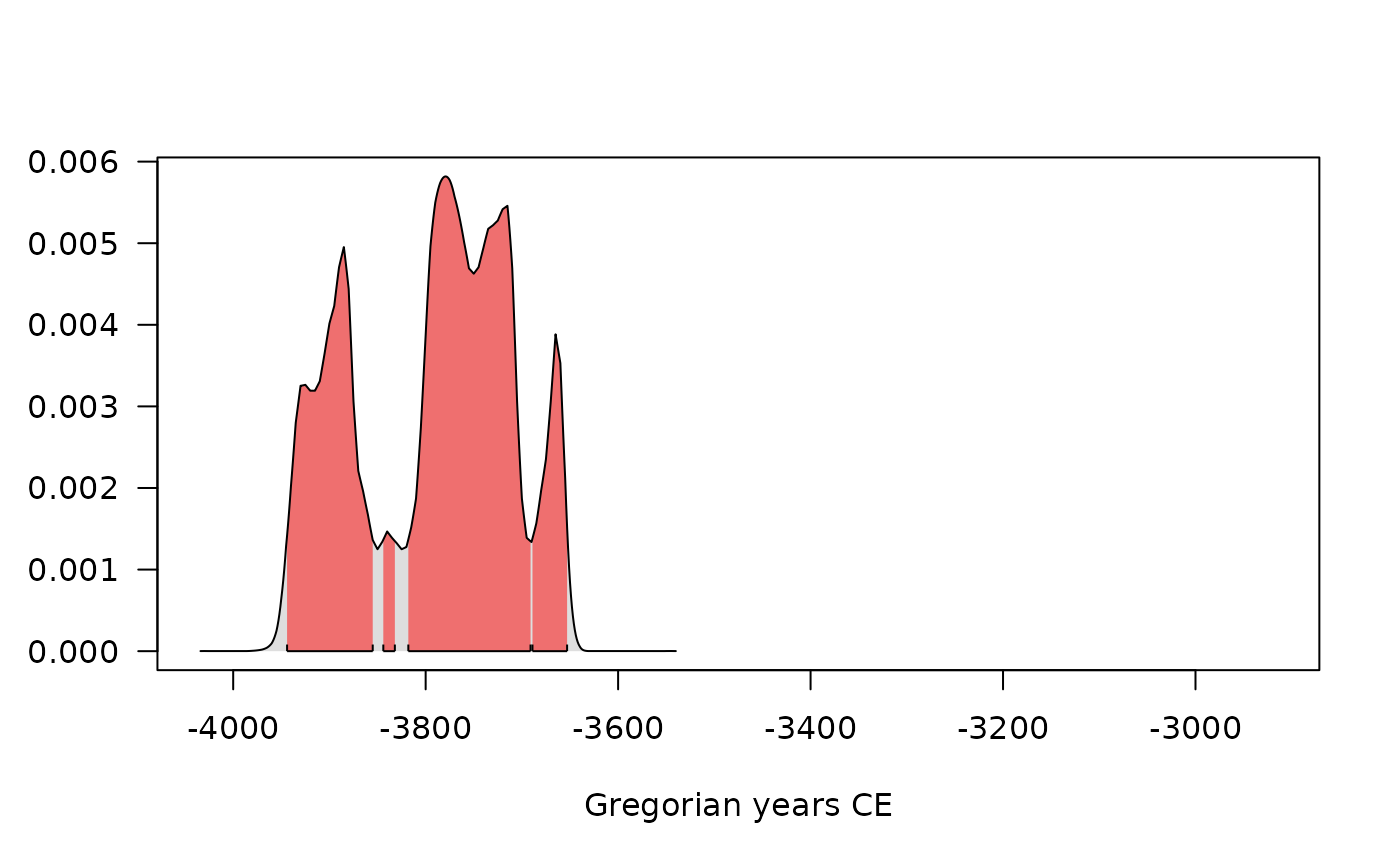# }